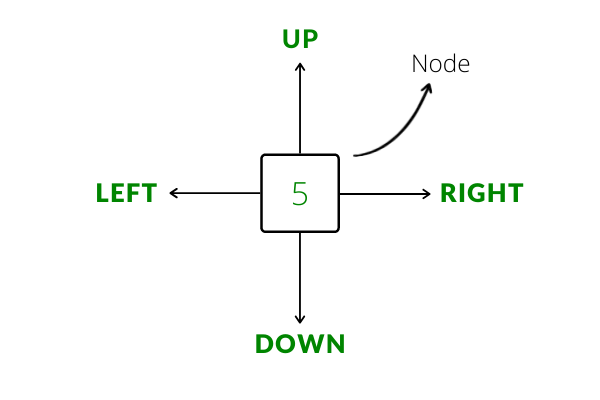# Orthogonal Linked List

• Difficulty Level : Medium
• Last Updated : 26 Sep, 2021

An Orthogonal Linked List is a data structure composed of fundamental elements called Nodes (similar to linked lists). Each node in an orthogonal Linked List points to 4 other nodes, namely up, down, left and right. In essence, just like a matrix is a 2D version of an array, an orthogonal linked list is a 2D version of a linear linked list.Algorithm to convert a matrix into an Orthogonal Linked List :

1. Create a node for each cell in the matrix. In a map, and also store the value of the cell and a pointer to the created node for the cell.
2. If the current row (i) is not the 0th row of the matrix, set the current node’s up pointer to the node of the cell just above it (use the map to get the correct pointer) and set the node above’s down pointer to the current node.
3. Similarly, if the current column (j) is not the 0the column of the matrix, set the current node’s left pointer to the node of the cell to the left of the current node and set the node to the left’s right pointer to the current node
4. repeat process 1 to 3 for every cell in the matrix
5. return map[matrix] to return the pointer to the top-left node of the orthogonal linked list

Below is an implementation of the algorithm above.

```Input:
matrix = {
{1, 2, 3},
{4, 5, 6},
{7, 8, 9}
}

Output:
A Node pointing to the top-left corner of the orthogonal linked list.

^      ^      ^
|      |      |
<--1 <--> 2 <--> 3-->
^      ^      ^
|      |      |
v      v      v
<--4 <--> 5 <--> 6-->
^      ^      ^
|      |      |
v      v      v
<--7 <--> 8 <--> 9-->
|      |      |
v      v      v```

## C++

 `#include ``using` `namespace` `std;` `struct` `MatrixNode``{``    ``int` `_val;``    ``MatrixNode* _u; ``// pointer to node above``    ``MatrixNode* _d; ``// pointer to node below``    ``MatrixNode* _l; ``// pointer to node towards left``    ``MatrixNode* _r; ``// pointer to node towards right` `    ``// Constructor for MatrixNode``    ``MatrixNode( ``int` `val = 0,``                ``MatrixNode* u = nullptr,``                ``MatrixNode* d = nullptr,``                ``MatrixNode* l = nullptr,``                ``MatrixNode* r = nullptr )``        ``{``            ``_val = val;``            ``_u = u;``            ``_d = d;``            ``_l = l;``            ``_r = r;``        ``}``};` `MatrixNode* BuildOrthogonalList(``int` `matrix[], ``int` `r, ``int` `c)``{``    ``// an unordered_map to store the {value, pointers} pair``    ``// for easy access while building the list``    ``unordered_map<``int``, MatrixNode*> mp;` `    ``for``(``int` `i = 0; i < r; i++)``    ``{``        ``for``(``int` `j = 0; j < c; j++)``        ``{``            ``// create a newNode for each entry in the matrix``            ``MatrixNode* newNode = ``new` `MatrixNode(matrix[i][j]);``            ``// store the pointer of the new node``            ``mp[matrix[i][j]] = newNode;` `            ``// set the up and down pointing pointers correctly``            ``if``(i != 0)``            ``{``                ``newNode->_u = mp[matrix[i - 1][j]];``                ``mp[matrix[i - 1][j]]->_d = newNode;``            ``}` `            ``// similarly set the left and right pointing pointers``            ``if``(j != 0)``            ``{``                ``newNode->_l = mp[matrix[i][j - 1]];``                ``mp[matrix[i][j - 1]]->_r = newNode;``            ``}``        ``}``    ``}` `    ``// return the start of the list``    ``return` `mp[matrix];``}` `void` `PrintOrthogonalList(MatrixNode* head)``{``    ``MatrixNode* curRow; ``// will point to the begin of each row``    ``MatrixNode* cur; ``// will traverse each row and print the element``    ``for``(curRow = head; curRow != nullptr; curRow = curRow->_d)``    ``{``        ``for``(cur = curRow; cur != nullptr; cur = cur->_r)``        ``{``            ``cout << cur->_val << ``" "``;``        ``}``        ``cout << endl;``    ``}``}` `int` `main()``{``    ``int` `matrix = {``        ``{1, 2, 3},``        ``{4, 5, 6},``        ``{7, 8, 9}``    ``};` `    ``MatrixNode* list = BuildOrthogonalList(matrix, 3, 3);``    ``PrintOrthogonalList(list);` `    ``return` `0;``}`

Application:
The most common application of orthogonal linked list is in sparse matrix representation. In brief, a sparse matrix is a matrix in which most of its elements are zeroes (or any known constant). They appear often in scientific applications. Representing sparse matrices as a 2D array is a huge wastage of memory. Instead, sparse matrices are represented as an orthogonal linked list. We create a node only for non-zero elements in the matrix and in each node, we store the value, the row index and the column index along with the necessary pointers to other nodes. This saves a lot of performance overhead and is the most memory-efficient way to implement a sparse matrix.

My Personal Notes arrow_drop_up